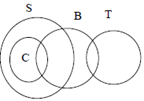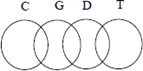# Robert Bosch Reasoning Question

1. 13,    52,    364,    4732,    ?
1. 67346
2. 78934
3. 52382
4. 75712
Here the series is written as the product of the number and the sum of the digits of that number
i.e. 13 × (3+1) = 52, 52 × (5+2) = 364, 364 × (3+6+4) = 4732, 4732 × (4+7+3+2) = 75712
DIRECTIONS for the question 2-3 : Read the information given below and answer the question that follows.

Six Teachers in a college have some constraints regarding the 6 sessions in a day. Darshana wants her session to be 3rd, which is immediately before lunch break and Prakash can’t take his session before lunch. Kundan runs home in between, so the management compelled him to take the last session. Sunny and his friend Rashmi are given sessions with a break in between their sessions. Priyanka doesn’t want her session after the break. Male and Female teachers are asked to take the sessions alternately.

2. Who will take the 5th session?
1. Sunny
2. Prakash
3. Rashmi
4. Darshana
1    2    3      4    5    6
Priy    Sun    Dars.     Prk    Rash.   Kun
So fifth session is taken by Rashmi
1. How many sessions are there in between Priyanka and Prakash? (Do not consider Break as a session.)
1. 1
2. 3
3. 2
4. 4
1    2    3      L    4    5    6
Priy    Sun    Dars.     Prk    Rash.   Kun
Clearly there are 2 sessions between Priyanka and Prakash
DIRECTIONS for the question 4-5 : These questions below have three statements followed by two conclusions numbered I and II. You have to take the given statements to be true even if they seem to be at variance with commonly known facts. Read all the conclusions and then decide which of the given conclusions logically follow from the given statements disregarding commonly known facts.
1. if neither conclusion follows
2. if conclusion II follows
3. if conclusion I follows
4. if both conclusions follow
2. Statements: All Cards are small. No Tree is small. Some Trees are Black
Conclusions: I. No card is Black.      II. No Card is a Treeor3. Statements: Some Cats are Good. No Cat is Dog. Some Dogs are Tall
Conclusions: I. Some Good are Tall      II. No Goods are Cats.

Conclusions I is ‘May be, May be not’ type and conclusion II is definitely wrong some cats are good. So none of them can be definitely concluded.orDIRECTIONS for the question 6-7 : Solve the following question and mark the best possible option.
4. In a certain language MITIGATE is written as MJVLKFZL. How will TERMINATE be written in the same code?
1. TFTPMSZGZ
2. TFUQNHTSZ
3. TFRSMSGZM
4. TFTPMSGAM
Here coding is done as M+0=M, I+1=J, T+2=V…so on
Hence for TERMINATE, coding will be T+0=T, E+1=F, R+2=T…..so on, giving us TFTPMSGAM
5. In a certain language ROLL is written as 9633. How will QUTT be written in the same code?
1. 7244
2. 8322
3. 8344
4. 9233
In ROLL, R=18, so write 1+8 = 9, O=15, so 1+5 = 6, L=12, so 1+2 = 3, and again L so again 3
So for QUTT, Q=17, so write 1+7 = 8, U=21, so 2+1 = 3, T=20, so 2+0 = 2 and again T=20 so again 2. Thus 8322
Meaningful 3 letter words starting with ‘A’ are ACT, ACE, AIR, ANT, ATE, ART
DIRECTIONS for the question 8-9 : Read the information given below and answer the question that follows.

Four friends analyze their height and weight and find that A is taller than B, who is heavier than only A. C is heavier than D, who is the tallest. B has the same rank in both the parameters of measurement. Here rank 1 is the heaviest and the tallest.

6. What are the positions of C in Height and Weight respectively?
1. 4 and 1
2. 4 and 2
3. 3 and 1
4. 3 and 2
B is heavier than only A, allows us to put B & A, 3rd and 4th. B has the same rank in ht and wt. and A is taller than B,
and C is heavier than D enables us to make this arrangement.
Rank (Ht.)   Name    Rank (Wt)    Name
1      D      1    C
2      A      2    D
3      B      3    B
4      C      4    A
Position of C in height is 4th while in weight is 1st
DIRECTIONS for questions 9-10: What should come in place of question mark (?) in the following number/alphabetic series?
7. What are the positions of A in Weight and Height respectively?
1. 4 and 1
2. 4 and 2
3. 3 and 1
4. None of these